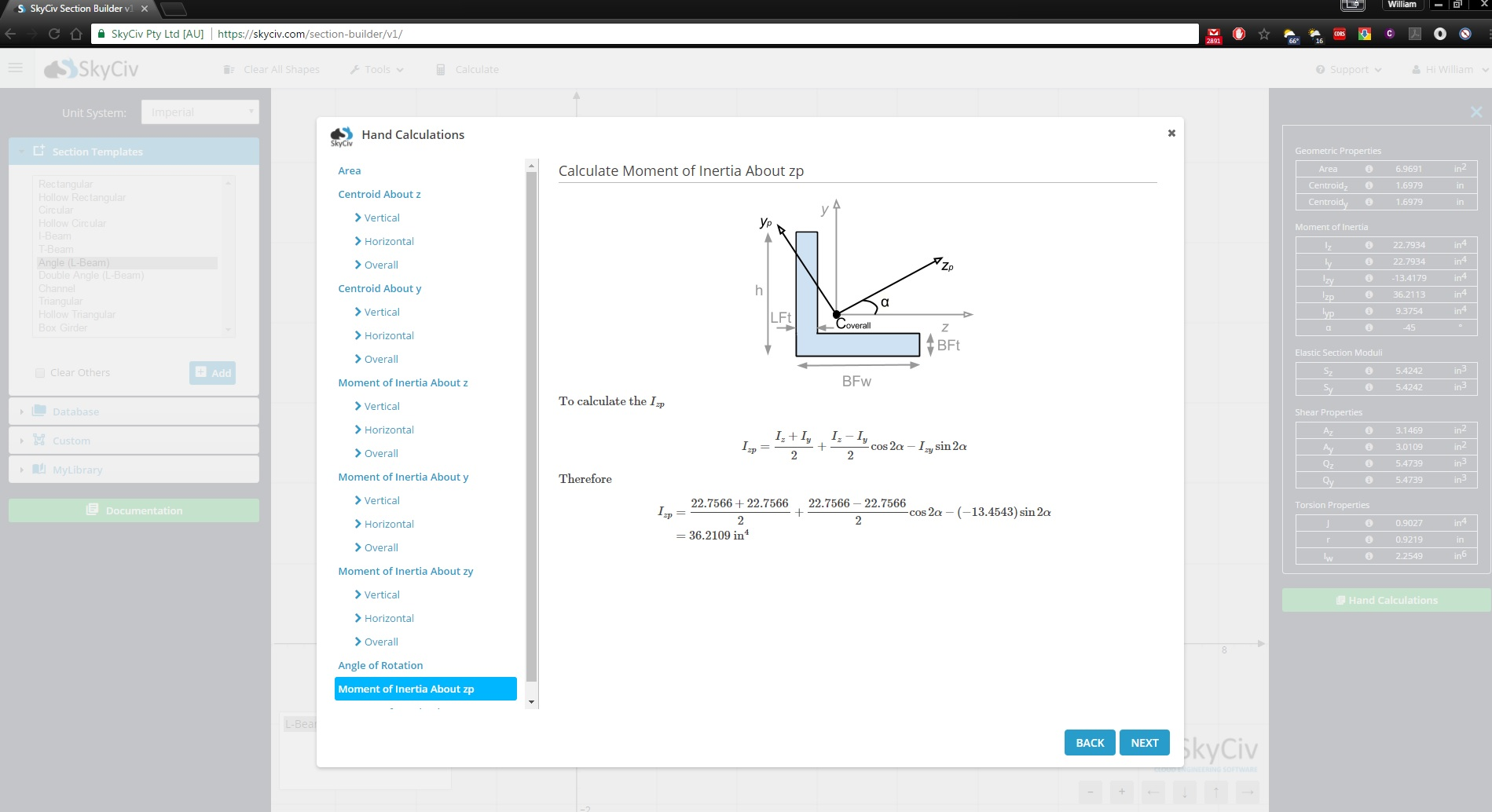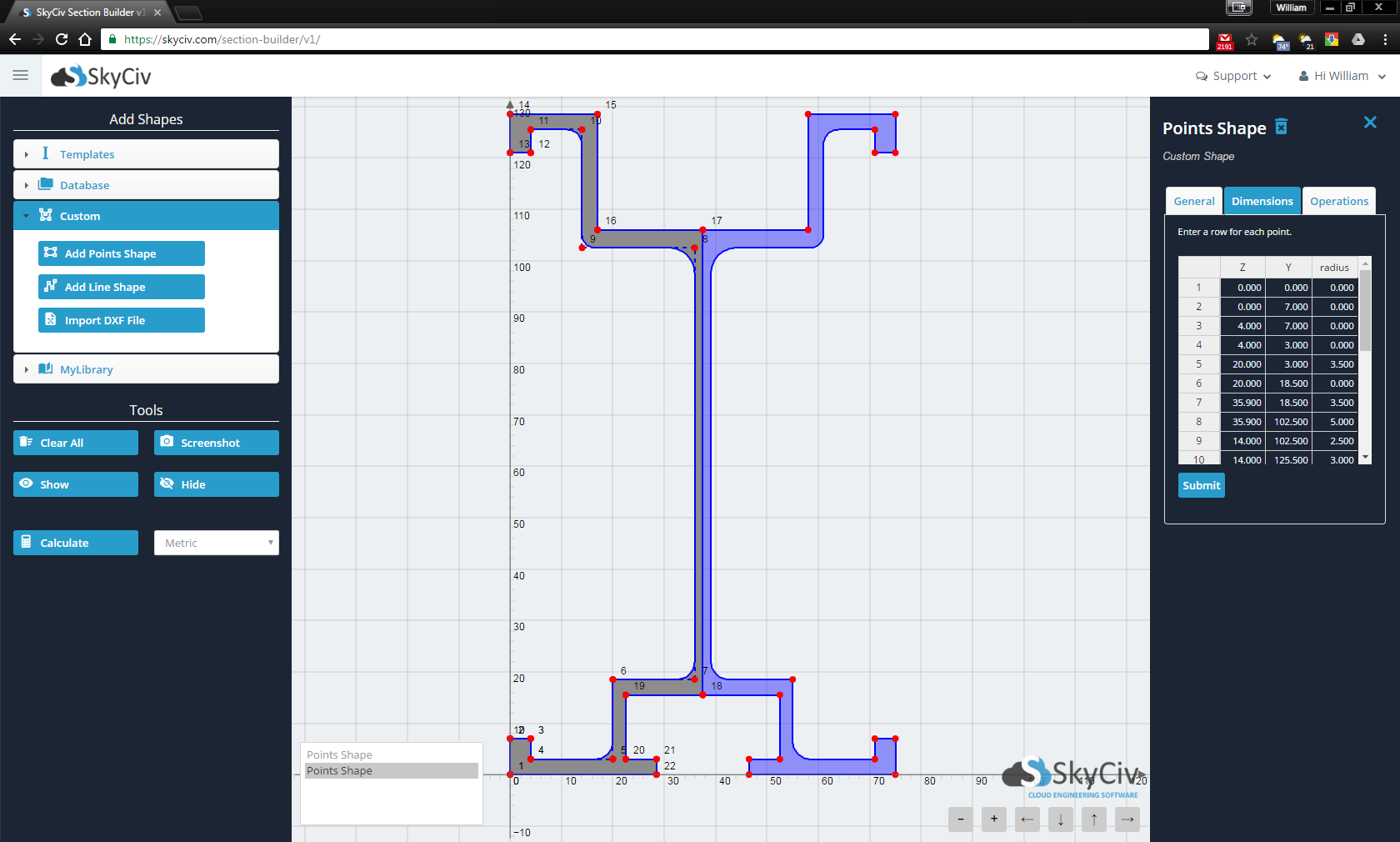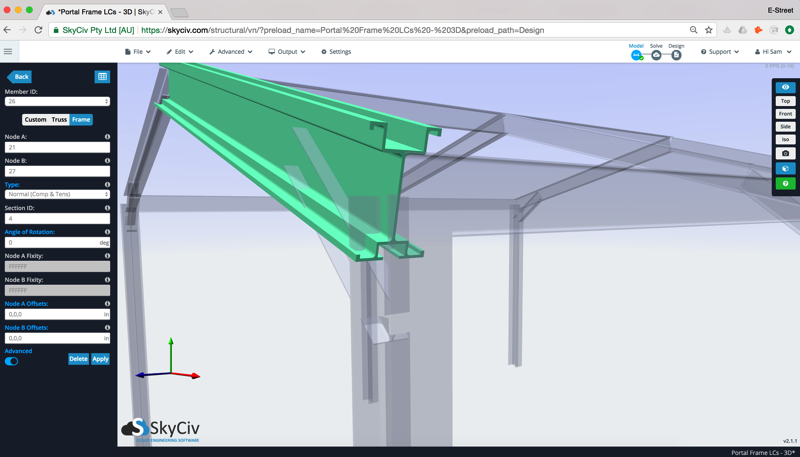# Free Moment of Inertia Calculator / Centroid Calculator

#### Calculate Moment of Inertia, Centroid, Section Modulus of Multiple Shapes

This free SkyCiv's Free Moment of Inertia Calculator is able to accurately and quickly calculate the moment of inertia among other section properties.

## How to use this Moment of Inertia Calculator?

To start using the free centroid calculator to calculate moment of inertia, simply choose the cross section you want to evaluate from the drop down list, then enter in the dimensions and click Calculate. The results for centroid, moment of inertia, statical moment section modulus and torsion constant will display on your right.

## Find the moment of inertia with ease

This free multi-purpose calculator is taken from our full suite Structural Analysis Software. It allows you to:

• Calculate the Moment of Inertia (I) of a beam section (Second Moment of Area)
• Centroid Calculator used to calculate the Centroid (C) in the X and Y axis of a beam section
• Calculate the First moment of area (Statical Moment of Inertia) (Q) of a beam section (First Moment of Area)
• A Section Modulus Calculator to calculate the Section Modulus (Z) of a beam section
• Calculate the Torsion Constant (J) of a beam section

## For more professional project needs:

To access the full functionalities of SkyCiv Structural Analysis Software, check out our professional plans. We have flexible plan options that tailor to different needs.b:
h:

Units:# Need More Functionality?### Hand Calculations

SkyCiv shows you how to calculate the moment of inertia by showing the step-by-step moment of inertia equations and calculations... The also show centroid and area calculations.### Custom and Built-Up Sections

SkyCiv Section Builder uses FEA to calculate key section properties, allowing you to model anything you need. Simply upgrade and get access to powerful software.### Integrated with Analysis Software

The SkyCiv Section Builder is fully integrated with all SkyCiv Analysis Software including SkyCiv Beam and SkyCiv Structural 3D - so you can analyze your section under loads.

## About the Moment of Inertia Calculator

This simple, easy-to-use moment of inertia calculator will find moment of inertia for a circle, rectangle, hollow rectangular section (HSS), hollow circular section, triangle, I-Beam, T-Beam, L-Sections (angles) and channel sections, as well as centroid, section modulus and many more results.

### Moment of Inertia

For instance, if you're looking how to calculate the moment of inertia of a rectangle you can use the tool above simply by selecting rectangle from the drop down list then entering some dimensions for height and width (e.g. 100, 200). After clicking "Calculate", the tool will calculate the moment of inertia. Our paid version will show the full hand calculations of how the tool got to this result. These detailed step-by-step instructions help for you to follow the calculations and learn how to calculate the moment of inertia. Alternatively, we have a tutorial on how to find moment of inertia.

### Section Modulus Calculator

The calculator will also produce results like section modulus (Z) and the statical moment of inertia (Q) for your sections. Finally, the result for Torsion Constant (J) will also display using this tool. The SkyCiv Section Builder will also produce these results accurately using FEA analysis of the cross section. So if you need more results, or to add custom shapes - please check out our extra functionality of the SkyCiv Section Builder.

### More Tools Available

SkyCiv also offers other tools such as I beam size tool and free structural design software. The dynamic section drawer will also show you a graphical representation of your beam section. So if you want to calculate the moment of inertia of a circle, moment of inertia of a rectangle or any other shapes, feel free to use the below software or our all-inclusive SkyCiv Section Builder.